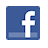Games
Problems
Go Pro!

Interest Compounded Continuously

Lesson Plans > Mathematics > Algebra > Functions > Logarithms

Slide Show

Click to show full screen

My investment increased by \$550 in 5 years, and by \$1200 in 10 years. What was my interest rate (compounded continuously)?

A = Pert

Interest Compounded ContinuouslyToday during class, I was working through a series of natural log problems with my pre-cal class. At one point I said, "I have an interesting problem I'd like to try. I don't know if I've ever done a problem quite like this before, so I don't actually know if we can solve it with the knowlege you have so far. Are you game to try it?" Surprisingly, they were willing to give it a try.

I enjoy doing this kind of exercise with my students once in awhile. It allows me to model for them problem solving skills. Sometimes I end up backed into a corner where I have to say, "I don't see a way to finish this problem - I think we're going to have to leave this here." Or, "This is getting way too ugly for us to finish now - I'll work out the rest of it at home, and report back tomorrow." But that, in itself, is a good lesson for students. It's okay to try something, even if you don't know it's possible. It gives students a better picture of mathematic exploration.

So today's curiosity question was: "My investment increased by \$550 in 5 years, and by \$1200 in 10 years. What was my interest rate (compounded continuously)?"

As we worked through this problem, I tried to let the students steer the direction we went, as much as possible. When they got stuck, I would suggest ideas that I noticed as possibilities. The solution took quite awhile to work through, but the result was quite satisfying. The solution the students came up with is below. I'll follow that up with a comment about something I noticed.

FIVE YEARS:
P + 550 = Pe5r
e5r =
P + 550
P

ln
P + 550
P
= 5r

TEN YEARS:
ln
P + 1200
P
= 10r

One of the students noticed that multiplying the first equation by 2 would result in two equations having 10r:

2ln
P + 550
P
= 10r = ln
P + 1200
P

Using the Qutotient Property:

2ln (P + 550) - 2ln P = ln FR(P + 1200) - ln P
2ln (P + 550) = ln (P + 1200) + ln P

Using both the Power Property (on the left) and the Product Property (on the right):

ln (P + 550)2 = ln P(P + 1200)

Thus, (P + 550)2 = P(P + 1200) *

P2 + 1100P + 302500 = P2 + 1200P
P = 3025

Now that we know P, we can plug that into one of our natural log equations to find r:

5r = ln
P + 550
P

5r = 0.16705
r = 0.0334 = 3.34%

* When we reached the point of
P + 550
P
= e5r and
P + 1200
P
= e10r, I pointed out that one equation was the square of the other, and it might be interesting to square the first one, and do a substitution. The students didn't want to go that route, because they thought it would be messy. Tomorrow I'm going to bring up the problem again, and show them what would have happened if they'd gone that route:

(P + 550)2
P2
= e10r =
P + 1200
P

(P + 550)2 = P(P + 1200)

This is the same as they obtained in the starred step above, with much fewer steps. It turned out that converting to log equations at that point in the problem was not the most efficient way of solving. Nevertheless, it did give them the result. I think it's important for students to see that there may be multiple ways of approaching the same problem.

Lesson by Mr. Twitchell

Featured Games on This Site

Match color, font, and letter in this strategy game
Trap all the dots in this problem-solving puzzle

Blogs on This SiteReviews and book lists - books we love!The site administrator fields questions from visitors.Like us on Facebook to get updates about new resources